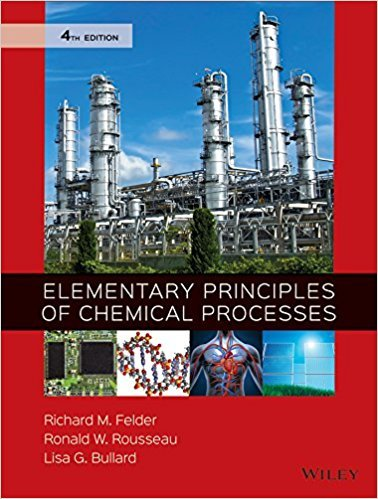×
×

# Solutions for Chapter 4.97: SUMMARY## Full solutions for Elementary Principles of Chemical Processes | 4th Edition

ISBN: 9780470616291Solutions for Chapter 4.97: SUMMARY

Solutions for Chapter 4.97
4 5 0 285 Reviews
18
1
##### ISBN: 9780470616291

This textbook survival guide was created for the textbook: Elementary Principles of Chemical Processes, edition: 4. Elementary Principles of Chemical Processes was written by and is associated to the ISBN: 9780470616291. This expansive textbook survival guide covers the following chapters and their solutions. Chapter 4.97: SUMMARY includes 1 full step-by-step solutions. Since 1 problems in chapter 4.97: SUMMARY have been answered, more than 46005 students have viewed full step-by-step solutions from this chapter.

Key Chemistry Terms and definitions covered in this textbook
• alkylthio group

An SR group.

• amplitude.

The vertical distance from the middle of a wave to the peak or trough. (7.1)

• anomers

Stereoisomeric cyclic hemiacetals of an aldose or ketose that differ from each other in their configuration at the anomeric carbon.

• atomic mass.

The mass of an atom in atomic mass units. (3.1)

• Basicity

An equilibrium property measured by the position of equilibrium in an acid-base reaction, as, for example, the acid-base reaction between ammonia and water.

• carbohydrates

Polyhydroxy aldehydes or ketones with molecular formula CxH2xOx.

• chelating agent

A polydentate ligand that is capable of occupying two or more sites in the coordination sphere. (Section 23.3)

• concentration

The quantity of solute present in a given quantity of solvent or solution. (Section 4.5)

• heat capacity

The quantity of heat required to raise the temperature of a sample of matter by 1 °C (or 1 K). (Section 5.5)

• intermediate

A structure corresponding to a local minimum (valley) in an energy diagram.

• intermolecular forces

The short-range attractive forces operating between the particles that make up the units of a liquid or solid substance. These same forces also cause gases to liquefy or solidify at low temperatures and high pressures. (Chapter 11: Introduction)

• mass defect

The difference between the mass of a nucleus and the total masses of the individual nucleons that it contains. (Section 21.6)

• Meta (m)

Refers to groups occupying 1,3-positions on a benzene ring.

• nanomaterial

A solid whose dimensions range from 1 to 100 nm and whose properties differ from those of a bulk material with the same composition. (Section 12.1)

• nitronium ion

The NO2+ ion, which is present in a mixture of nitric acid and sulfuric acid.

• Organometallic compound

A compound that contains a carbon-metal bond.

• partial pressure

The pressure exerted by a particular gas in a mixture. (Section 10.6)

• potential energy

The energy that an object possesses as a result of its composition or its position with respect to another object. (Section 5.1)

A compound such as a phenol that selectively reacts with radicals to remove them from a chain reaction and terminate the chain

• reaction quotient (Q)

The value that is obtained when concentrations of reactants and products are inserted into the equilibrium expression. If the concentrations are equilibrium concentrations, Q = K; otherwise, Q ? K. (Section 15.6)

×